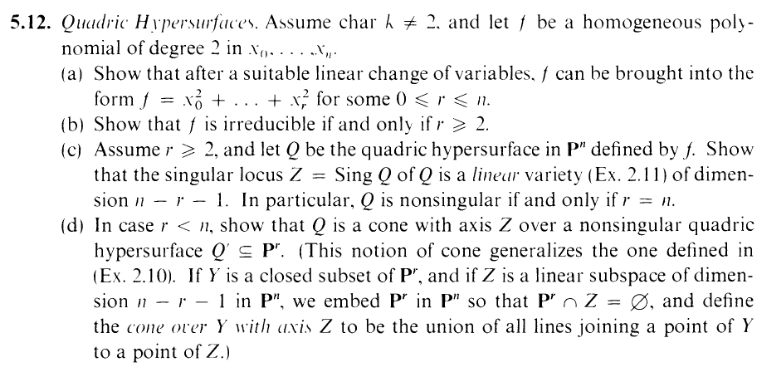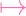I.5.12d

8/26/2021It's okay they'll just get counted as covid deaths LOLOLOLOLOL I have a list here of some of the fallen readers of this blog: Rainbow Dash, Pinkie Pie, Twilight Sparkle, Lee Goldson, TK-47, Sakurafish (extra rip), the boymoder poster, Juan Ovus (your sacrifice will never be forgotten. Just remember that although Ovyu wasn't your biological brother, he still loved you), Kilim, Ronald McDonald, his sociopathic friend... The list keeps going. And they're only some of the 6 million we lost. Many of them are nameless anons, just like (You). Now, everyone, kneel. #KneelForNeil Kneel for the lost souls."Ummmm, was it that bad?" Errrr, not as bad as 3.16 (that one only took an hour to be beaten off the face of this blog), but bad enough. OK: although redacted, I did in fact complete a,b,c (and this time I do have a copy of it, in case I ever need to look back at the math part, LOL). So let's finish the job. Here we are, baby: Part D.

### DSo... the thing is, I can do this exercise. Just watch. The "Q" they're looking for is obviously Q= Z(f) (it's just f in k[x0,,xr] instead of k[x0,xn]. Now, let's say P = (p0,,pr) Q and S = (s0,,sr,sr+1,,sn) Z (and we know s0,,sr = 0). So if I embed Pr in Pn like they describe. Then I'm sending

 (x0,…,xr)(x0,…,xr, 0, 0,…, 0)

(so clearly Pr Z = , as needed). So where does P get sent to? P gets sent to (p0,,pr, 0, 0,, 0), right? So we have

 P = (p0,…,pr, 0,…, 0) S = (0,…, 0,sr+1,…,sn)0

Now, we are going to connect P and S with a line. Now, if you did read yesterday's awful post, you'll know that I don't know how to do this in projective space. However, let's take the "common sense" route and connect them parametrically as if they were in affine space. The "line" between the two is the set of points L = (l0,,ln), where

 l0 = s0 + (p0 - s0)tln = sn + (pn - sn)t

Plugging in the 0s, we get

 l0 = p0tlr = prt lr+1 = sr+1(1 - t)ln = sn(1 - t)

where t k is a scalar. Now keep in mind that there are no restrictions on sr+1,,sn are free, which means, according to the equations above, lr+1,,ln are also FREE. So I can get rid of those equations entirely. Now we are left with

 l0 = p0tlr = prt

But WAIT: This set of equations say that l0,,lr merely have the same restrictions as p0,,pr. I.e. f(l0,,lr) = 0. I.e. f(l0,,ln) = 0.

The only problem is that I used the parametric representation of a line, which is not how we defined "lines" in the context of varieties. A line in projective space is a linear variety–the zero set of a set of linear homogenous polynomials. I still don't know how to construct that. I know it's equivalent to the parametric representation BUT AAAAAAAAAAAAA.